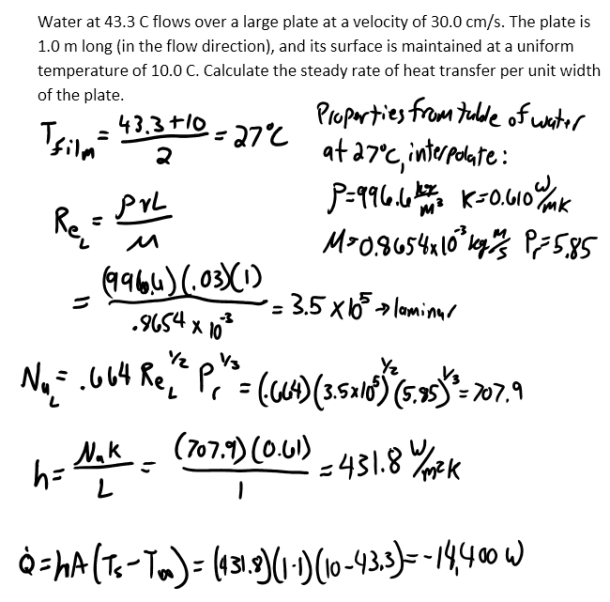heat and mass transfer problems engineering equations heat and mass transfer school homework engineering heat and mass transfer formulas heat and mass transfer solutions to heat and mass transfer problems full solution engineering problem solution heat and mass transfer math problems engineering equations heat and mass transfer school homework engineering solutions to heat and mass transfer formulas heat problems mass problem solutions to transfer problems full solution heat and mass transfer
heat and mass transfer problems engineering equations heat and mass transfer school homework engineering heat and mass transfer formulas heat and mass transfer solutions to heat and mass transfer problems full solution engineering problem solution heat and mass transfer math problems engineering equations heat and mass transfer school homework engineering solutions to heat and mass transfer formulas heat problems mass problem solutions to transfer problems full solution heat and mass transfer
Highalphabet Home Page heat and mass transfer problem solutions Heat and Mass Transfer Page
Water at 43.3 C flows over a large plate at a velocity of 30.0 cm/s. The plate is 1.0 m long (in the flow direction), and its surface is maintained at a uniform temperature of 10.0 C. Calculate the steady rate of heat transfer per unit width of the plate.Water at 43.3 C flows over a large plate at a velocity of 30.0 cm/s. The plate is 1.0 m long (in the flow direction), and its surface is maintained at a uniform temperature of 10.0 C. Calculate the steady rate of heat transfer per unit width of the plate.